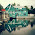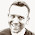## poniedziałek, 23 lutego 2015

### Javascript Array Methods - Slice(), Splice(), Map(), Reduce(), ForEach(), Some(), Every(), IndexOf(), Concat()

Recently, I have noticed that I have problems with remember the difference betwean JS method `Array.prototype.slice` and `Array.prototype.splice`, so I decided for learning purposes to write this short post as a reference about JS array methods. In this post I included few commonly methods:

## slice()

`Slice` method create new array which containes shallow copy of elements from original array.This method does not change original array.

Syntax: `array.slice(begin, end)`

`Begin` parameter is the index of element from where the copy process starts. `Slice` copy element until it reach the `end`, wherein element on index `end` is not copied into new array. When parameter `begin` or `end` is negative, index of the element is counted from the end of the array.

``````var array = 'lukasz-szewczak'.split('');
console.log(array.slice(0,2))
// ["l", "u"]
console.log(array.slice(0,-2))
// ["l", "u", "k", "a", "s", "z", "-", "s", "z", "e", "w", "c", "z"]
console.log(array.slice(-8))
//["s", "z", "e", "w", "c", "z", "a", "k"]
``````

We can use `slice` method to convert array like object into array. Very often you can meet wih calling of this method inside function to converte `arguments` object into array.

``````function list() {
return Array.prototype.slice.call(arguments, 0);
}
list(1, 2, 3);
// [1, 2, 3]
``````

Instead of `Array.prototype.slice` we can use `[].slice.call`;

## splice()

`Splice` change the original array by removing or adding new elements

Syntax: `array.splice(start, deleteCount, item1, item2, ...)`

`Start` parameter define index from which array will be changed, negative value mean that searching start from the end of the array, `deleteCount` describes how many elements are to be removed from the array.`ItemN` describes the element to be added to the array. Method returns array of deleted elements.

``````var array = [1, 2, 3, 9, 10];
var removed = array.splice(3, 0, 4, 5, 6, 7, 8);
console.log(array)
// [1, 2, 3, 4, 5, 6, 7, 8, 9, 10]
console.log(removed)
// []
removed = array.splice(3,5);
console.log(array)
// [1, 2, 3, 9, 10]
console.log(removed)
// [4, 5, 6, 7, 8]
``````

## map()

The `map` method transforms the array by applying a function to all of its elements and building a new array from the returned values. The new array will have the same length as the input array.

Syntax: `array.map(callback)`

Callback function which generates new array element is invoke with this three arguments:

• currentValue - current element from original array
• index - index of current element
• orgArr - original array on which transformation is made
``````var array = [1, 2, 3, 4];
var plusOne = array.map(function(el, index, orgArr) {
return el + 1;
});
console.log(plusOne);
//[2, 3, 4, 5]
``````

## reduce()

`Reduce` is one of the method I ignored for a long time, because I don't fuly understand it, but my attitiude changed after I started reading a book Eloquent Javascript.

The `reduce` method is used to calculate a single value from an array.

Syntax: `array.reduce(callback, initialValue)`

`reduce` executes `callback` function once for each element in the array, which receives four arguments:

• previousValue - value from previous invocation of the callback function or `initialValue` if supplied
• currentValue - current element from the array
• index - index of the current element
• array - the array `reduce` was called upon
``````var numbers = [12,34,23,56,78,34];
function max(array){
function mathMax(a, b) {return Math.max(a, b);}
return array.reduce(mathMax);
}

function avarage(array){
function plus(a, b) {return a+b;}
return array.reduce(plus) / array.length;
}
console.log(max(numbers));
//78
console.log(avarage(numbers));
//39.5
``````

And here is another example of `reduce`, where I change array into the list object using `reduce` method. (This is my result for exercise from the book Eloquent Javascript)

``````function arrayToList(arr){
return arr.reverse().reduce(function(previousVal, currentVal){
if (previousVal){
return {
value: currentVal,
rest:previousVal
};
}
else {
return {
value: currentVal,
rest: null
};
}
},null);
};
console.log(arrayToList([10,20,30,40,50,60]));
``````

## forEach()

The standard method (from ES5) to iterate over array. Thanks to this method code is shorter and cleaner, there is probably one small problem that is you cannot break this method using `break` keyword like the classical for loop (http://stackoverflow.com/questions/6260756/how-to-stop-javascript-foreach). The `forEach` method takes one argument whis is function to excecuted on every element.

Syntax: `array.forEach(callback)`

Callback function takes three arguments:

• currentValue – current element being processed
• index – index of the current element
• array
``````[10,20,30,40,50,60].forEach(function (element, index) {
console.log('Element: {', element, '}, index {', index,'}');
});
// Element: { 10 }, index { 0 }
// Element: { 20 }, index { 1 }
// Element: { 30 }, index { 2 }
// Element: { 40 }, index { 3 }
// Element: { 50 }, index { 4 }
// Element: { 60 }, index { 5 }
``````

## some()

If You want to check wheater at least one element in array meets your specific criteria you can use method `some` which will return `true` as soon as it find element meeting this specific criteria.

Syntax: array.some(callback)

`callback` is a function to test if an element meets our criteria. Callback function takes three arguments:

• currentValue – current element being processed
• index – index of the current element
• array
``````function ifElementBiggerThen30(element){ return element > 30;}
[10,20,30,40,50,60].some(ifElementBiggerThen30);
//true
``````

## every()

On the other hand, if You want to check if all elements in array meet yours specific criteria you can use method `every` which will return `false` as soon as it find element which does not meet your criteria.

Syntax: array.every(callback);

`callback` is a function to test if an element meets our criteria. Callback function takes three arguments:

• currentValue – current element being processed
• index – index of the current element
• array
``````[10,20,30,40,50,60].every(Number.isInteger);
//true
[10,20,30,40,'test',60].every(Number.isInteger);
//false
``````

## indexOf()

Next method can help you find all occurence of element in array

Syntax: `array.indexOf(searchElement, fromIndex)`

`searchElement` is of course element we are looking for in the array, if element is founded then method returs it index or -1 if no element is found. `indexOf` compares element with `searchEelement` using strict equality ( === )

``````[10,20,30,40,50,60,10,20,40].indexOf(10);
//0
[10,20,30,40,50,60,10,20,40].indexOf(10,1);
//6
[10,20,30,40,50,60,10,20,40].indexOf(70);
//-1
``````

## concat()

If you want to join value(s) or arrays with existing array we can use `concat` method which joins all provided values with array on which it was called upon and return new array.

Syntax: `array.concat(value1, value2, ...)`

Where parameter `valueN` can be an array or not;

``````var some_array = [1,2,4,6,'a','b','c'],
new_array = [10,9,8,7,'e','d','c'].concat(some_array,'D');
console.log(new_array);
//[10, 9, 8, 7, "e", "d", "c", 1, 2, 4, 6, "a", "b", "c", "D"]
``````

Writing this reference helped me a lot to consolidate my knowledge about the above-mentioned array methods. Now I will go to check new ES6 arrays method. :-)

#### 2 komentarze:

1.Very good article

1.Thank you :-)